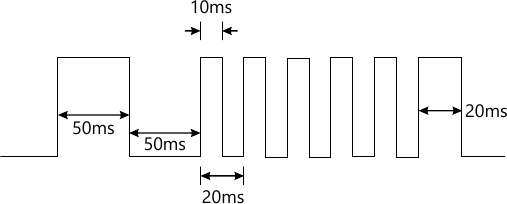MORE IN Microcontroller for Embedded Systems
VTU Electronics and Communication Engineering (Semester 4)
Microcontroller for Embedded Systems
June 2015
Total marks: --
Total time: --
INSTRUCTIONS
(1) Assume appropriate data and state your reasons
(2) Marks are given to the right of every question
(3) Draw neat diagrams wherever necessary

1 (a) Compare 8051, 8052 and 8031 microcontroller.
5 M
1 (b) Explain the internal RAM section of 8051 μc with required diagrams.
10 M
1 (c) For the following μc ICS, determine the ROM memory address of AT89C51 with 4KB, DS89C420 with 16 KB and DS5000 with 32KB.
5 M

2 (a) What are the merits and demerits of indirect addressing mode?
5 M
2 (b) State the type of addressing mode used for the following instructions:
ii) CJNE A, #29h, AGHAIN
iii) [email protected] R0
iv) XCH A, R3
v) CLR C,
5 M
2 (c) Explain the working of DAA instruction with an example. Assume that data is 99h and 99h.
5 M
2 (d) Write a program to convert hexadecimal number to decimal. Include suitable comments.
5 M

3 (a) Write a program to load accumulator with the value 55h and complement the content of accumulator 900 times.
5 M
3 (b) For AT89C51, with a crystal frequency of 22 MHz, write a program to generate a delay of 5ms.
5 M
3 (c) Explain the working of JZ LABEL instruction with an example. Is zero flag present in 8051?
5 M
3 (d) Explain the calculation of checksum byte in ROM with an example.
5 M

4 (a) Explain the feature of ADC 0804. Also explain the working of its various pins.
10 M
4 (b) Explain the principle of stepper motor. Write a program to rotate motor 64° in clockwise direction. The motor has step angle of 2°. Write the 4 step sequence also. The motor has steps per revolution = 180, number of rotor teeth=45, movement per 4 step sequence = 8°.
10 M

5 (a) Explain the bit status of TMOD special function resister. Also, explain its various modes.
5 M
5 (b) Using P1.5 timer-1 in mode-1, write a program to generate the following waveform as shown in Fig. Q5 (b). Assume that system clock is 11.0592 Mhz. Show the delay calculations. This waveform should be generated continuously.10 M
5 (c) Write a 'C' program that continuously gets a single bit of data from P1.7 and sends it to P1.0 while simultaneously creating a square wave of 200 μs period on pin P2.5. Use timer-0 to create the square wave. Assume that crystal is 11.0592 Mhz.
5 M

6 (a) Explain the bit status of SCON special function register.
5 M
6 (b) Write a 'C' program for 805 to transfer the letter 'A' serially at 4800 baud continuously. Use 8-bit data and 1 stop bit. Use timer 1 in mode 2.
5 M
6 (c) Determine the baud rate if THI= -2, SMOD=1, XTAL=11.0592 MHz. Is this baud rate supported by IBM PCS?
5 M
6 (d) Calculate the control words of 8255 for the following cases:
i) All the ports A, B and C are output ports (mode-0)
ii) PA= in, PB=out, PCL=out=PCH.
5 M

7 (a) Explain the expansion of MSP μc. Also explain how MSP μc is different from conventional μc, with an example.
8 M
7 (b) Explain the difference between MSP430XIXX, MSP430F2XX, MSP430X3XX, MSP430X4XX and MSP430x μcs.
8 M
7 (c) Explain the salient features of MSP430μC.
4 M

8 (a) Explain the functions of watchdog timer, basic timer - 1, real time clock, timer A and timer B in MSP430μc.
10 M
8 (b) Explain the interfacing of LCD to MSP430μc.
10 M

More question papers from Microcontroller for Embedded Systems